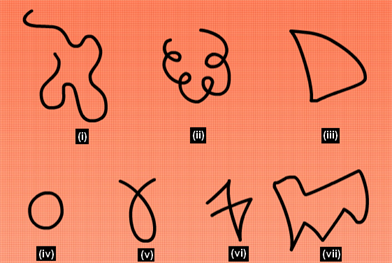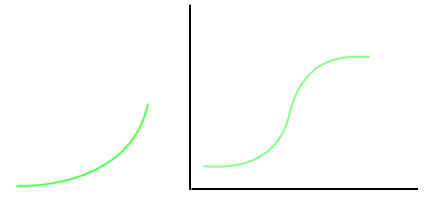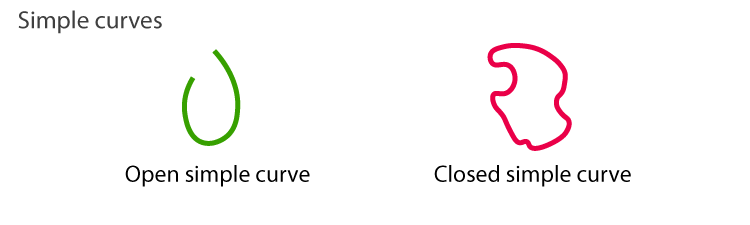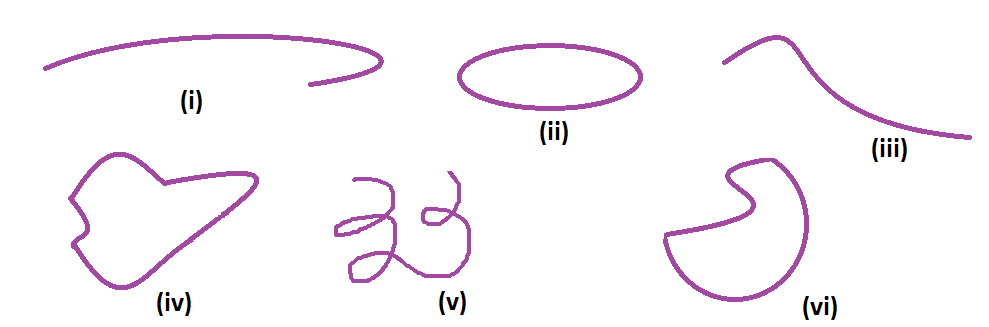# Curved Line

## Definition

A curved line is the one that is not straight and is bent. If the curvature is not zero, it is considered as a curve line. Ideally, it is smooth and continuous.

### Curved line imagesYou see curves everywhere around you. Be it art or decoration or a general thing, and curves can be seen around you. Curves were initially called as lines. To clear the concept of line and curve, they started calling line as a straight line. Now the straight line and curve are different.

It is mostly used in the graphical representation of functions. It is one of the vital topics in Math.### Difference Between Straight and Curved Line

 Straight Line Curved Line A straight line is the shortest line which joins any two points. It always moves in one direction. A bent line which is not straight is called Curved Line. It doesn’t move in one direction.

### Examples of curved lines

There are many examples of curved lines like the alphabets – C and S. Whereas the letters A, M, N, L, etc are not the examples of curves since they can be formed by joining the line segments (or straight lines).### Types of Curved Line

• Algebraic Curve
• Transcendental Curve

Algebraic Curve – A plane curve where a set of points are located on the Euclidean plane and are represented in terms of polynomials is called Algebraic Curve. The polynomial’s degree denotes the degree of the curve.

C = {(a, b) ∈ R2: P(a, b) = 0}

Transcendental Curve – This curve is different from the algebraic curve. This curve might have many intersecting points which will be straight. These might also have an infinite number of inflexion points. It is not a polynomial in a and b.

### Practice Question

Question: Identify the open and closed curves from the below figure.## Frequently Asked Questions – FAQs

### What is a curved line?

A curved line is a type of straight line with bent or in other words, it is a geometrical object similar to the line having curvature.

### What are the examples of curved lines?

There are many examples of curved lines in the English alphabet such as C, S and O.

### Why we use curved lines?

Curved lines are used mainly in the graphical representation of different types of functions.

### What are the types of curves?

There are two types of curves namely simple open curves and simple closed curves. For example, “C” is the simple open curve and “O” is the simple closed curve that we can see in alphabets.

### What is a simple curve?

A curve that doesn’t cross itself is called a simple curve, otherwise, it is a complex (or non-simple) curve. For example, “U” is the simple curve and “8” is the non-simple curve.